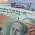Saturday, 9 June 2012

The ASX 200 Cyclically Adjusted PE (aka ASX 200 PE10 or ASX200 CAPE) – June 2012 Update

The ASX 200 closed on Friday priced at 4,063 which is a 12% fall from 1 year ago.  At this price I have earnings (which are 12-month trailing underlying profits) at 337 which results in a price earnings ratio (P/E Ratio) of 12.1.  In comparison I have calculated the S&P 500 P/E Ratio at 14.3 and the FTSE 100 P/E Ratio at 9.4 this month.

The history of the ASX 200 P/E Ratio since 1993 can be seen in my first chart today along with the Ratio I am personally far more interested in which is the ASX 200 PE10 (effectively  an ASX 200 cyclically adjusted PE or ASX 200 CAPE for short).  The method is based on that made famous by Professor Robert Shiller and in this instance it is simply the ratio of Inflation Adjusted Monthly ASX 200 Monthly Prices to Inflation Adjusted Average Earnings.  Today the ASX 200 PE10 sits at 13.9.  A full summary of relevant ASX 200 PE10 data follows:
• ASX 200 PE10 = 13.9
• Dataset Average PE10 = 22.1.  If this average was “fair value” then it indicates that today the ASX200 is 37% undervalued.  I’m not convinced of this though and think it is a result of a relatively short dataset but I’ll talk more of that later in this post.
• Dataset Median PE10 = also at 22.1
• Dataset 20th Percentile = 16.9
• Dataset 80th Percentile = 27.5
My second chart plots ASX 200 inflation adjusted Earnings and Dividends.  Both continue to rise nicely from the 2010 lows with Earnings at 337 and Dividends at 210.  That gives us a current dividend yield of around 5.2% which doesn’t seem to shabby.

My third chart shows why I use the ASX200 PE10 ratio as part of my private retirement investing today strategy.  This chart shows the ASX200 Price vs the Nominal 5 Year Nominal Total Return.   The correlation is -0.46 with an R^2 of 0.22.  With the ASX200 PE10 at 13.9 the chart trendline implies a future Nominal 5 Year Total Return of 86%.  Even if I adjust this metric for inflation (not charted today) the trendline implies a real return of 69%.

Finally, one problem this data has is that it is quite a short set.  For my own investments I therefore now try and correct for this by making quite a large assumption, which is that there is a high correlation between Australian Equities and International Equities performance.  With this assumption I then look at my mature S&P 500 dataset which tells me that since 1881 the average PE10 has been 16.4 and from 1993 it has averaged 26.6.  Taking a ratio of these two PE10’s and multiplying by the current ASX200 Average PE10 I arrive at a “pseudo long run” Average PE10 of 13.6.  Comparing that with today’s PE10 of 13.9 suggests the market is pretty much fairly valued today and it is that data that I am making my own personal investment decisions on.

As always DYOR.

Assumptions include:
• All historic figures are taken from official data from the Reserve Bank of Australia.
• Latest ASX200 P/E ratio is calculated using the 08 June 2012 Price and the May 2012 Earnings which are extrapolated from the RBA’s published P/E.
• June 2012 price is the 08 June 2012 market close.
• June 2012 Earnings and Dividends are assumed to be the same as the May numbers.
• Inflation data from April, May and June 2012 is extrapolated.

1.Great data thanks for posting. The R2 on the equation is low though, would.be interesting to either add more variables to see if this could increase, or reduce some of.the outliers?

2.Hi Matt

Thanks for stopping by. It could partly be due to the small dataset. Eyeballing the chart shows a lot of data points already forming a nice linear correlation. It's the otliers that sit north of the trend between a PE10 of about 22 and 28 that seem to cause the problem. You might have just given me an idea for another post. What dates are those outliers?

That said if I look at the other PE10's that I track:
- S&P 500 5 Year Total Return R^2 = 0.2093
- FTSE 100 5 Year Capital Gain R^2 = 0.1645
shows the S&P 500 which I have data from since 1881 as having a slightly worse R^2.

Cheers
RIT